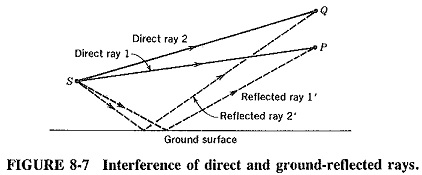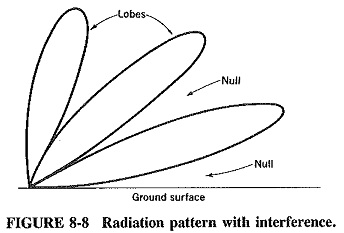## Interference of Electromagnetic Waves:

Interference of Electromagnetic Waves occurs when two waves that left one source and travelled by different paths arrive at a point. This happens very often in high-frequency sky-wave propagation and in microwave space-wave propagation. It occurs when a microwave antenna is located near the ground, and waves reach the receiving point not only directly but also after being reflected from the ground. This is shown in Figure 8-7.It is obvious that the direct path is shorter than the path with reflection. For some combination of frequency and height of antenna above the ground, the difference between paths 1 and 1′ is bound to be exactly a half-wavelength. There will thus be complete cancellation at the receiving point P if the ground is a perfect reflector and partial cancellation for an imperfect ground.

Another receiving point, Q, may be located so that the path difference between 2 and 2′ is exactly one wavelength. In this case reinforcement of the received waves will take place at this point and will be partial or total, depending on the ground reflectivity. A succession of such points above one another may be found, giving an Interference of Electromagnetic Waves pattern consisting of alternate cancellations and reinforcements. A pattern of this form is shown in Figure 8-8.The curve of Figure 8-8 joins points of equal electric intensity. The pattern is due to the presence of an antenna at a height above the ground of about a wavelength, with reflections from the ground (assumed to be plane and perfectly conducting) causing Interference of Electromagnetic Waves.

A pattern such as the one shown may be calculated or plotted from actual field-strength measurements. The “flower petals” of the pattern are called lobes. They correspond to reinforcement points such as Q of Figure 8-7, whereas the nulls between the lobes correspond to cancellations such as P of Figure 8-7.

At frequencies right up to the VHF range, this Interference of Electromagnetic Waves will not be significant because of the relatively large wavelengths of such signals. In the UHF range and above, however, interference plays an increasing part in the behaviour of propagating waves and must definitely be taken into account. It is certainly of great significance in radar and other microwave systems.

For instance, if a target is located in the direction of one of the null zones, no increase in the transmitted radar power will make this target detectable. Again, the angle that the first lobe makes with the ground is of great significance in long-range radar. Here the transmitting antenna is horizontal and the maximum range may be limited not by the transmitted power and receiver sensitivity, but simply because the wanted direction corresponds to the first null zone. It must be mentioned that a solution to this problem consists of increasing the elevation of the antenna and pointing it downward.

Scroll to Top